Courses

# Simplification MCQ 2

## 20 Questions MCQ Test Quantitative Techniques for CLAT | Simplification MCQ 2

Description
This mock test of Simplification MCQ 2 for Quant helps you for every Quant entrance exam. This contains 20 Multiple Choice Questions for Quant Simplification MCQ 2 (mcq) to study with solutions a complete question bank. The solved questions answers in this Simplification MCQ 2 quiz give you a good mix of easy questions and tough questions. Quant students definitely take this Simplification MCQ 2 exercise for a better result in the exam. You can find other Simplification MCQ 2 extra questions, long questions & short questions for Quant on EduRev as well by searching above.
QUESTION: 1

Solution:
QUESTION: 2

Solution:
QUESTION: 3

###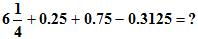Solution:
QUESTION: 4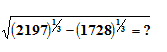Solution:
QUESTION: 5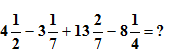Solution:
QUESTION: 6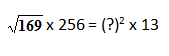Solution:
QUESTION: 7

What should come in the place of question mark (?) in the following equation?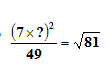Solution:

The correct option is C.
(7*x)2/49 = _/81
⇒ 49*x2/49 = _/81
⇒ x2 = _/81
⇒ x2 = 9
⇒ x = 3

QUESTION: 8

Simplify: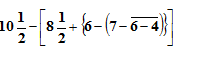Solution:
QUESTION: 9

(If x = 4, y = 3, then the value of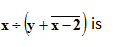Solution:
QUESTION: 10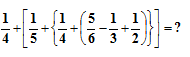Solution:
QUESTION: 11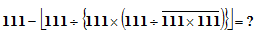Solution:
QUESTION: 12

Find the value of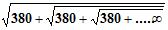Solution: Of courseyou seelet x = the problemnow take square both sides ..x^2-x-380=0as putting x=20
QUESTION: 13

Which one of the following fractions is the greatest?

Solution:
QUESTION: 14

The sum of the digits of a two-digit number is 8. If the digits are reversed, the number is decreased by 54. Find the number.

Solution:
QUESTION: 15

The sum of the digits of a two-digit number is 8. If the digits are reversed, the number is decreased by 54. Find the number.

Solution:
QUESTION: 16

If the sum of a number and its square is 156, what is the number?

Solution:
QUESTION: 17

What is the number in the unit place in (5427)641?

Solution:

The correct answer is B as the unit place will be 7 only .

QUESTION: 18

A number when divided by 1092 gives a remainder 60. What remainder would be obtained by dividing the same number by 28?

Solution: In these type of of questions we apply a trick by just dividing the given remainder by given no.
60÷28
remainder will come( 4)
QUESTION: 19

The sum of squares of two numbers is 85 and the square of first number is 77 more than by the square of second number. The product of the two numbers is

Solution:
QUESTION: 20

Find the number in the unit place in (321)321 x (325)326.

Solution:

321 raised to 321=1 in unit digit and any number ending in 5 raised to any number has always 5 as unit digit, so 1×5=5